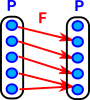# Maths - Poset

A poset can be represented by a directed graph where there is 0 or 1 (but not more than one) arrows between the nodes.

For example if we have a relation between two elements 'p' and 'q':

 p >= q Then we write an arrow p->q

## Properties

See Wikipedia

#### Non-strict partial order

• reflexivity: a ≤ a (every element is related to itself.)
• antisymmetry: if a ≤ b and b ≤ a then a = b
• transitivity: if a ≤ b and b ≤ c then a≤c.

A non-strict partial order is also known as an antisymmetric preorder.

#### Strict partial order

• Irreflexivity: not a < a (no element is related to itself)
• Transitivity: if a < b and b < c then a < c ,
• Asymmetry: if a < b then not b < a.

A strict partial order is also known as a strict preorder.

## Isotone Maps

An isotone map preserves the ordering.

## Fixpoint and W-completeness

W-complete - each well-ordered subset of P has a supremum.

A partially ordered set P is W-complete iff each selfmap of P has a fixpoint.

That is W-completeness implies a fixpoint and a fixpoint implies W-completeness.

 To get an intuitive feel try to construct a map without a fixpoint. The top element cant map to itself so let it go to the element below. The next element down cant map to the top element since an isotone map must preserve the ordering. So again it must map to (at least) the element below. If we continue like this then, sooner or later, we will run out of elements. So there must be at least one fixpoint.